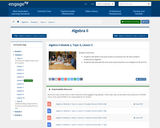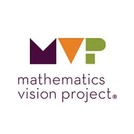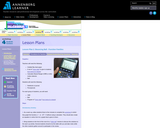Updating search results...

# 189 Results

View
Selected filters:
• Math 2Conditional Remix & Share Permitted
CC BY-NC-SA
Rating
0.0 stars

Students develop facility with graphical interpretations of systems of equations and the meaning of their solutions on those graphs. For example, they can use the distance formula to find the distance between the centers of two circles and thereby determine whether the circles intersect in 0, 1, or 2 points.
By completing the squares, students can convert the equation of a circle in general form to the center-radius form and, thus, find the radius and center. They can also convert the center-radius form to the general form by removing parentheses and combining like terms.
Students understand how to solve and graph a system consisting of two quadratic equations in two variables.

Subject:
Math 1
Math 2
Mathematics
Material Type:
Lesson
Author:
EngageNY
02/23/2020Conditional Remix & Share Permitted
CC BY-NC-SA
Rating
0.0 stars

Students understand the possibility that an equation—or a system of equations—has no real solutions. Students identify these situations and make the appropriate geometric connections.

Subject:
Math 2
Mathematics
Material Type:
Lesson
Author:
EngageNY
02/23/2020Conditional Remix & Share Permitted
CC BY-NC-SA
Rating
0.0 stars

Students solve quadratic equations with real coefficients that have complex solutions. They recognize when the quadratic formula gives complex solutions and write them as a + bi for real numbers a and b.

Subject:
Math 2
Mathematics
Material Type:
Lesson
Author:
EngageNY
02/23/2020Conditional Remix & Share Permitted
CC BY-NC-SA
Rating
0.0 stars

Students solve quadratic equations with real coefficients that have complex solutions. Students extend polynomial identities to the complex numbers.
Students note the difference between solutions to the equation and the x-intercepts of the graph of said equation.

Subject:
Math 2
Mathematics
Material Type:
Lesson
Author:
EngageNY
02/23/2020Conditional Remix & Share Permitted
CC BY-NC-SA
Rating
0.0 stars

Students understand the Fundamental Theorem of Algebra; that all polynomial expressions factor into linear terms in the realm of complex numbers. Consequences, in particular, for quadratic and cubic equations are understood.

Subject:
Math 2
Math 3
Mathematics
Material Type:
Lesson
Author:
EngageNY
02/23/2020Conditional Remix & Share Permitted
CC BY-NC-SA
Rating
0.0 stars

Students will define sine and cosine as functions for all real numbers measured in degrees.
Students will evaluate the sine and cosine functions at multiples of 30 and 45.

Subject:
Math 2
Mathematics
Material Type:
Lesson
Author:
EngageNY
02/23/2020Rating
0.0 stars

Using tiles to represent variables and constants, learn how to represent and solve algebra problem. Solve equations, substitute in variable expressions, and expand and factor. Flip tiles, remove zero pairs, copy and arrange, and make your way toward a better understanding of algebra.

Subject:
Math 1
Math 2
Math 3
Mathematics
Material Type:
Interactive
Provider:
National Council of Teachers of Mathematics
Author:
Illuminations
02/26/2019Conditional Remix & Share Permitted
CC BY-NC-SA
Rating
0.0 stars

This is a ten question assessment&nbsp;afer a lesson taught on angle relationships and parallel lines.

Subject:
Math 2
Material Type:
Assessment
Author:
KORI ELLIOTT
07/14/2020Conditional Remix & Share Permitted
CC BY-NC-SA
Rating
0.0 stars

The purpose of this task is to allow students to use their prior understandings of similar triangles to develop an understanding of trigonometric ratios. As the discussion about the trigonometric ratios and their usefulness surfaces, be sure to name the ratios with their names (sine, cosine, tangent) and demonstrate how they are most frequently written as cos, sin, tan.

Subject:
Math 2
Mathematics
Material Type:
Lesson
Author:
The Mathematics Vision Project
03/12/2020Conditional Remix & Share Permitted
CC BY-NC-SA
Rating
0.0 stars

The purpose of this task is for students to connect the completing the square procedure learned in the previous two tasks to graphing parabolas. The task asks students to complete the square to change the equation of a quadratic function in standard form into vertex form. Students will need to extend their understanding of completing the square in an expression to an equation to maintain the equality, requiring that they add and subtract an equivalent term to one side of the equation (effectively adding zero) or that they add the same thing to both sides of the equation. After getting the equation into vertex form, students graph the equation of the parabola.

Subject:
Math 2
Mathematics
Material Type:
Lesson
Author:
The Mathematics Vision Project
03/11/2020Conditional Remix & Share Permitted
CC BY-NC-SA
Rating
0.0 stars

The purpose of this task is for students to solidify their understanding about inverse functions. Students will understand how to find the inverse of a function and know when to restrict the domain so that they can produce an invertible function from a non-invertible function. Students will also become familiar with square root functions as a result of this task and will connect square root functions to their domain, range, and graphs.

Subject:
Math 2
Mathematics
Material Type:
Lesson
Author:
The Mathematics Vision Project
03/11/2020Rating
0.0 stars

Susie is organizing the printing of tickets for a show. She has collected prices from several printers. Your task is to use a table and algebra to advise Susie on how to choose the best printer.

Subject:
Math 1
Math 2
Math 3
Mathematics
Material Type:
Activity/Lab
Assessment
Lesson Plan
Provider:
MARS, Mathematics Assessment Resource Service
Author:
Shell Center Team
02/26/2019Conditional Remix & Share Permitted
CC BY-NC-SA
Rating
0.0 stars

The purpose of this task is for students to solidify their understanding of piecewise functions using their background knowledge of domain and linear functions. Students will solidify their understanding of piecewise functions by

• answering questions relating to a piecewise function
• using their knowledge of domain to graph each section of the piecewise function
• graphing complete piecewise-defined functions from equations
• interpreting the context of a piecewise function

Subject:
Math 2
Mathematics
Material Type:
Lesson
Author:
The Mathematics Vision Project
03/11/2020Rating
0.0 stars

Students explore quadratic functions by using a motion detector known as a Calculator Based Ranger (CBR) to examine the heights of the different bounces of a ball. Students will represent each bounce with a quadratic function of the form y = a (x - h)^2 + k. This lesson plan is based on the activity Tremain Nelson uses in the video for Part II of this workshop.

Subject:
Math 1
Math 2
Math 3
Mathematics
Material Type:
Lesson Plan
Provider:
Annenberg Learner
Author:
Annenberg Learner
02/26/2019Rating
0.0 stars

In this lesson, students explore relationships between x-intercepts, factors, and roots of polynomial functions using the graphing calculator. Students also investigate rational functions, identifying the roots and the asymptotes as well as holes in the graphs. Students construct boxes of various dimensions using graph paper, collect height and volume data, and create a scatterplot in order to determine the height of the box with the maximum volume. Students can solve this problem using a graphing calculator or by using their own scatterplots drawn by hand. The use of questioning by the teacher, and the group work of the students are important features of this lesson.

Subject:
Math 1
Math 2
Math 3
Mathematics
Material Type:
Activity/Lab
Lesson Plan
Provider:
PBS
Author:
PBS Teachers Mathline
02/26/2019Conditional Remix & Share Permitted
CC BY-NC-SA
Rating
0.0 stars

The purpose of this task is to develop students’ understanding of the procedure of completing the square using area models. In the task, students will use diagrams of area models to make sense of the terms in a perfect square trinomial and discover the relationship between coefficients of a quadratic expression. They will use this understanding to complete the square to find equivalent forms of quadratic expressions. In this task, the quadratic expressions will be limited to those in which the coefficient of the !! term is 1. This task is the beginning of a learning cycle that ends in students using the completing the square procedure to find the vertex form of a quadratic function and graph the associated parabola.

Subject:
Math 2
Mathematics
Material Type:
Lesson
Author:
The Mathematics Vision Project
03/11/2020Educational Use
Rating
0.0 stars

Students learn a more formal definition of conditional probability and are asked to interpret conditional probabilities. Data are presented in two-way frequency tables, and conditional probabilities are calculated using column or row summaries.

Subject:
Math 2
Mathematics
Material Type:
Interactive
Provider:
EngageNY
Author:
EngageNY
04/04/2014Conditional Remix & Share Permitted
CC BY-NC-SA
Rating
0.0 stars

In Math 8 students came to understand that a two-dimensional figure is congruent to another if the second can be obtained from the first by a sequence of rotations, reflections, and translations. In this task students are given two congruent figures and asked to describe a sequence of transformations that exhibits the congruence between them. While exploring potential sequences of transformations, students will notice how corresponding parts of congruent figures have to be carried onto one another, and they may look for ways that this can be accomplished in a consistent sequence of steps.

Subject:
Math 2
Mathematics
Material Type:
Lesson
Author:
The Mathematics Vision Project
03/10/2020Conditional Remix & Share Permitted
CC BY-NC-SA
Rating
0.0 stars

In this task students are asked to develop a strategy for locating the center of a rotation, which leads to the observation that the center of rotation lies on the perpendicular bisectors of the segments joining image/pre-image pair of points. Such segments are defined to be chords of the circles on which the image/pre-image points are located. This work provides a method for finding the center of a circle. Students should also be able to prove that the perpendicular bisector of a chord contains a diameter of the circle and that the central angle formed by the radii that pass through the endpoints of the chord is bisected by the perpendicular diameter.

Subject:
Math 2
Mathematics
Material Type:
Lesson
Author:
The Mathematics Vision Project
03/12/2020Conditional Remix & Share Permitted
CC BY-NC-SA
Rating
0.0 stars

The purpose of this task is to practice thinking through and contributing to the reasoning of others when working through a written geometric proof. Students will also practice all of the proof techniques discussed in this module: reasoning from a diagram; organizing a logical chain of reasoning from the given statements to the conclusion of the argument; and drawing upon postulates, definitions and previously proved statements to support the chain of reasoning. Three theorems are proved in this task:
• The medians of a triangle meet at a point.
• The angle bisectors of a triangle meet at a point.
• The perpendicular bisectors of the sides of a triangle meet at a point.

It is not necessary for students to create these theorems from scratch, but they should be able to follow and extend the reasoning of the arguments presented, based on the diagrams and previously proved theorems. The ideas proved here are novel and somewhat surprising. These theorems should demonstrate the power of deductive reasoning to establish the validity of ideas that at first seem counterintuitive.

Subject:
Math 2
Mathematics
Material Type:
Lesson
Author:
The Mathematics Vision Project